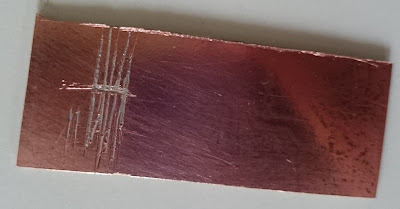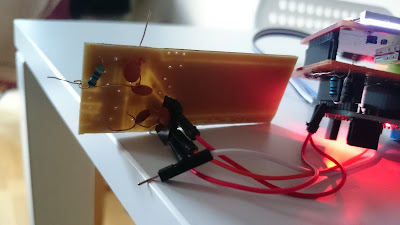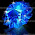## Sunday, October 25, 2015

### Simple AD8307 rf power meter for sweeper using ad9850 dds and arduino for testing crystals etcSweeping a 14.318 crystal on the dds sweeper
After finishing with a simple vswr plotter using an arduino pro mini and a tft, i decided to improve the rf measurement section of the analyser with an ad8307 based power rf meter. The desig is simple and is popular among hobbyists. I got hold of an ad8307 in smd form, too small for me. So the challenge was to try some ugly construction using a piece of copperclad. Ideaa is to keep a large ground plane by leaving the entire copper board as ground. It was pretty easier than my guess. The idea is to use a sharp engraving knife to make small lines and islands of copper to be used as solder points.  So no etching as i used in my previous post (Salt water etching for a simple pcb).

So first i drew some lines on the copper board to allow the smd ad8307 pins to be soldered. The ugly board is shown in the following figures.lines drawn on the copper clad for the ad8307
and here is how it looks after finishing upfinished rf power measuring board
and the whole board with arduinorf power meter with arduino
And the schematic is hereSchematic for rf power meter with ad8307

To generate a sweep signal, i used the same code as in my antenna /vswr plotter and the voltage output from ad8307 is read by an analogue pin on the atmega328 and plotted on the tft. Some of figures from sweeping a crystal at 14.318 mhz is shown below

Next step is to integrate in to the swr bridge and use it as a vswr meter. I will soon  update with the code, once it is finished. Thanks for visiting

Read more on the progress: https://www.riyas.org/2017/04/a-compact-swr-analyser-and-wspr-signal-generator.html

Test snippet for ad8307 power meter (connect the output voltage from ad8307 to A1 pin or PC1 on atmega) and hook up an LCD shield

```//Sample using LiquidCrystal library
#include <LiquidCrystal.h>
#include <OneWire.h>
LiquidCrystal lcd(8, 9, 4, 5, 6, 7);

// define some values used by the panel and buttons
int lcd_key     = 0;
#define btnRIGHT  0
#define btnUP     1
#define btnDOWN   2
#define btnLEFT   3
#define btnSELECT 4
#define btnNONE   5

long result;
// Read 1.1V reference against AVcc
ADMUX = _BV(REFS0) | _BV(MUX3) | _BV(MUX2) | _BV(MUX1);
delay(2); // Wait for Vref to settle
result = 1126400L / result; // Back-calculate AVcc in mV
return result;
}

void setup()
{

Serial.begin(9600); // initialize serial communication at 9600 bits per second:

lcd.begin(16, 2); // set up the LCD's number of columns and rows:
lcd.setCursor(5, 0); // (note: line 1 is the second row, since counting begins with 0):
lcd.print("LB7UG"); // Print a message to the LCD.
lcd.setCursor(1, 1); // (note: line 1 is the second row, since counting begins with 0):
lcd.print("RF-Power meter"); // Print a message to the LCD.
delay(4000); //Delay 4 sek
lcd.clear(); //clear display
//analogReference(INTERNAL);

}

void loop()
{
float bvoltage=readVcc()/1000.0;//get the vcc voltage rail.
float voltage = sensorValue * (bvoltage / 1023.0);  // Convert the analog reading (which goes from 0 - 1023) to a voltage (0 - 5V):

//double dBm=(voltage-2.1808f)/0.025f; // calculate dBm
double dBm=((voltage-2.160f)/0.025f)+20; // calculate dBm and add20 for attenuator

double Pu= pow( 10.0, (dBm+30) / 10.0); //pwr in uW
double Pm= pow( 10.0, (dBm) / 10.0); //pwr in mW
double Pw= pow( 10.0, (dBm-30) / 10.0); //pwr in W
Serial.print(voltage); Serial.print("V");
Serial.print(dBm);Serial.println("dBm");
lcd.setCursor(0, 0);
lcd.print(dBm);lcd.print("dBm");
lcd.setCursor(0, 1);
Serial.print(Pm); Serial.println("mW"); // print mW
lcd.print(Pm);lcd.print("mW"); // print mW on LCD
PrintPu :
if (dBm <= 0)  Serial.print(Pu); if (dBm <= 0)  Serial.println("uW"); // print uW
if (dBm <= 0)  lcd.print(Pu); if (dBm <= 0)  lcd.print("uW"); // print uW
PrintPw :
if (dBm >= 30) Serial.print(Pw); if (dBm >= 30) Serial.println("W");// print W
if (dBm >= 30) lcd.print(Pw); if (dBm >= 30) lcd.print("W");// print W
lcd.setCursor(10, 1);
lcd.print(sensorValue);
lcd.setCursor(10, 0);
lcd.print(voltage);
delay(500);
lcd.clear();

}
```

1.I've just ordered the lcd..waiting,waiting.
Looking forward to your updated code for vswr.
de john VK5COR

2.I stumbled across your project looking at ideas for the AD8307 as s-meter and decided why not do the swr bridge too. I have a QRP SWR Bridge from Kits and Parts.
http://kitsandparts.com/bridge.php

73, Michael
KM4OLT

1.Thanks Michael for the feedback. AD8307 is a nice/old device with a good dynamic range. The bridge on kitsnparts looks nice and can be used together with ad8307 and an atmega328 to form a digital swr/pwr meter. Good luck wirh your experiments

73s Riyas

3.Hi. The LCD screen is 1.8 inch OLED or 1.8 inch Nextion TFT or etc..... ????

4.Hi. The LCD screen is 1.8 inch OLED or 1.8 inch Nextion or etc.... which one? Thank you for your answers.